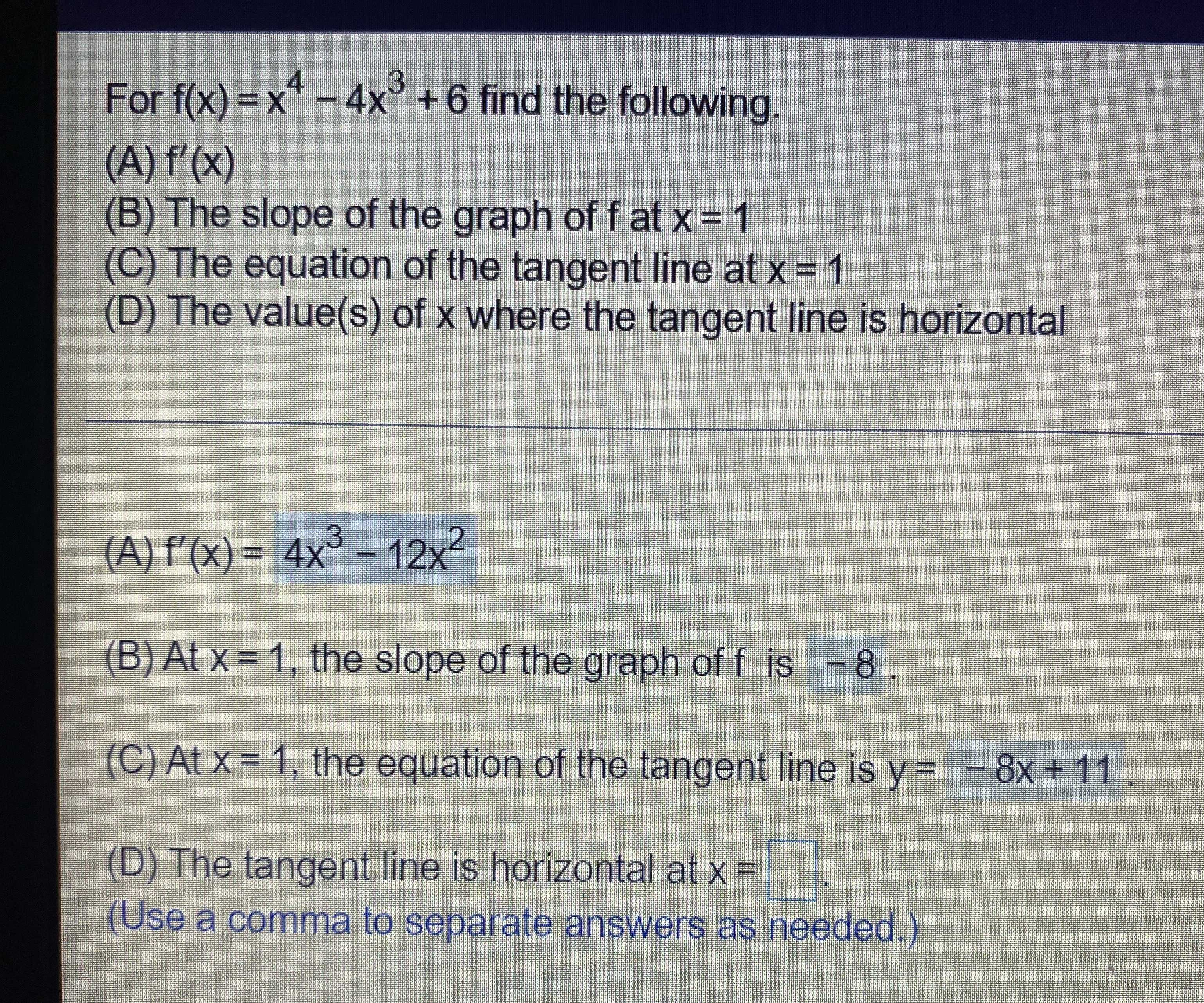### Still have math questions?

Algebra
QuestionFor $$f ( x ) = x ^ { 4 } - 4 x ^ { 3 } + 6$$ find the following. (A) $$f ^ { \prime } ( x )$$

(B) The slope of the graph of $$f$$ at $$x = 1$$ (C) The equation of the tangent line at $$x = 1$$ (D) The value(s) of $$x$$ where the tangent line is horizontal (A) $$f ^ { \prime } ( x ) = 4 x ^ { 3 } - 12 x ^ { 2 }$$

(B) At $$x = 1$$ , the slope of the graph of $$f$$ is $$- 8 .$$ (C) At $$x = 1$$ , the equation of the tangent line is $$y = - 8 x + 11$$ . (D) The tangent line is horizontal at $$x = \square$$

(Use a comma to separate answers as needed.)

(a) 4x$$^{3}$$ - 12x$$^{2}$$